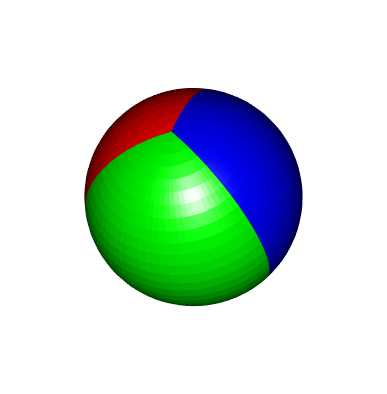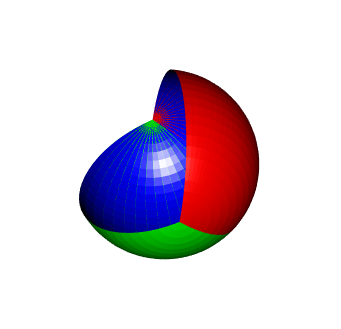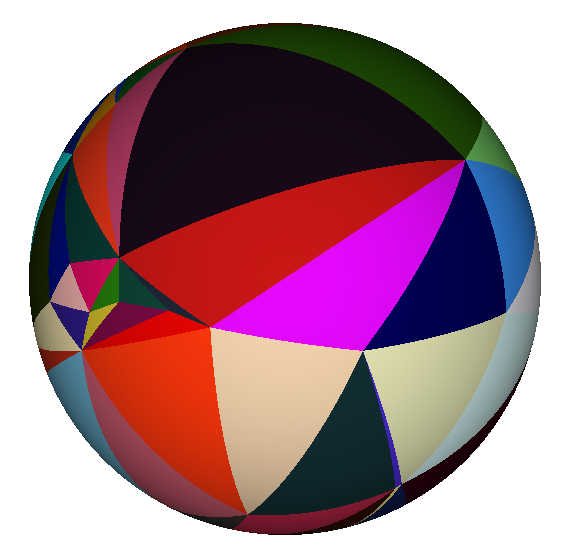# Drawing spherical wedges with rgl

Posted on February 22, 2018 by Stéphane Laurent
Tags: R, graphics

This post provides some code to draw a sphere wedge with the R library rgl.

As an helper function, I use spherical2cartesian to convert from spherical coordinates to Cartesian coordinates:

spherical2cartesian <- function(rthetaphi){
r <- rthetaphi
theta <- rthetaphi
phi <- rthetaphi
c(x=r*cos(theta)*sin(phi), y=r*sin(theta)*sin(phi), z=r*cos(phi))
}

Now, here is the main function:

sphereWedge <- function(theta1, theta2, radius=1, nm=30, np=30, col="blue"){
thetas <- seq(theta1, theta2, length.out=nm)
phis <- pi * 1:(np-1)/np
meridians <- sapply(phis,
function(phi) sapply(thetas,
simplify=FALSE)
for(i in 1:(np-2)){
x1 <- plyr::aaply(meridians[[i]], 2, spherical2cartesian)
x2 <- plyr::aaply(meridians[[i+1]], 2, spherical2cartesian)
for(j in 1:(nm-1)){
spherical2cartesian(meridians[][,j+1])), color=col)
spherical2cartesian(meridians[[np-1]][,j+1])), color=col)
}
}
}

A simple example:

sphereWedge(0, pi/3, col="purple")Now let’s draw a tricolor sphere:

sphereWedge(0, 2*pi/3, col="red")
sphereWedge(2*pi/3, 4*pi/3, col="green")
sphereWedge(4*pi/3, 2*pi, col="blue")As you can see, I use 2*pi and not 0 for the blue wedge. That should be equivalent. However, and I don’t know why, using 0 instead of 2*pi colors the interior of the two other wedges:

sphereWedge(0, 2*pi/3, col="red")
sphereWedge(2*pi/3, 4*pi/3, col="green")
sphereWedge(4*pi/3, 0, col="blue")## Spherical triangles with rgl

I also did a code which draws spherical triangles for rgl. I won’t reproduce it here. If you are interested, it is available in this gist.